/

### Value-at-Risk

The Value-at-Risk, VaR, of a portfolio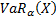is the outcome (loss),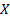, that will be exceeded on a fraction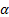of occasions. It requires a time-scale (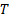) as well as a confidence level.

Where the support of the distribution is continuous, the Value-at-Risk with confidence levelis: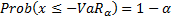If the distribution is unbounded below then this means that if the probability density function of the distribution is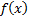we have: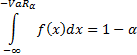If the lower bound is finite, e.g.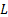then the definition would replace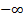with.

Note: sometimesand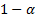are interchanged or losses are deemed positive rather than negative etc.

Visually the difference between VaR and Tail VaR (TVaR) may be seen in either of the following charts: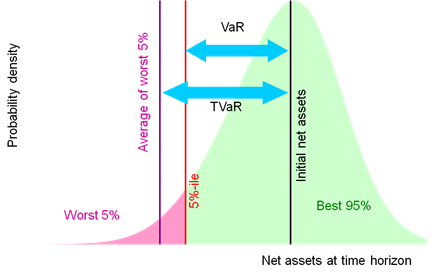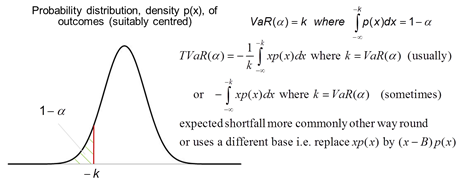VaR is not (in general) a coherent risk measure, whilst TVaR is. VaR is arguably more shareholder focused and TVaR more regulator/customer focused, see VaR versus TVaR mindsets.

If several different risk exposures are contributing to the overall VaR then it often becomes important to identify the contribution each is making to the total. This can be done using marginal Value-at-Risk.